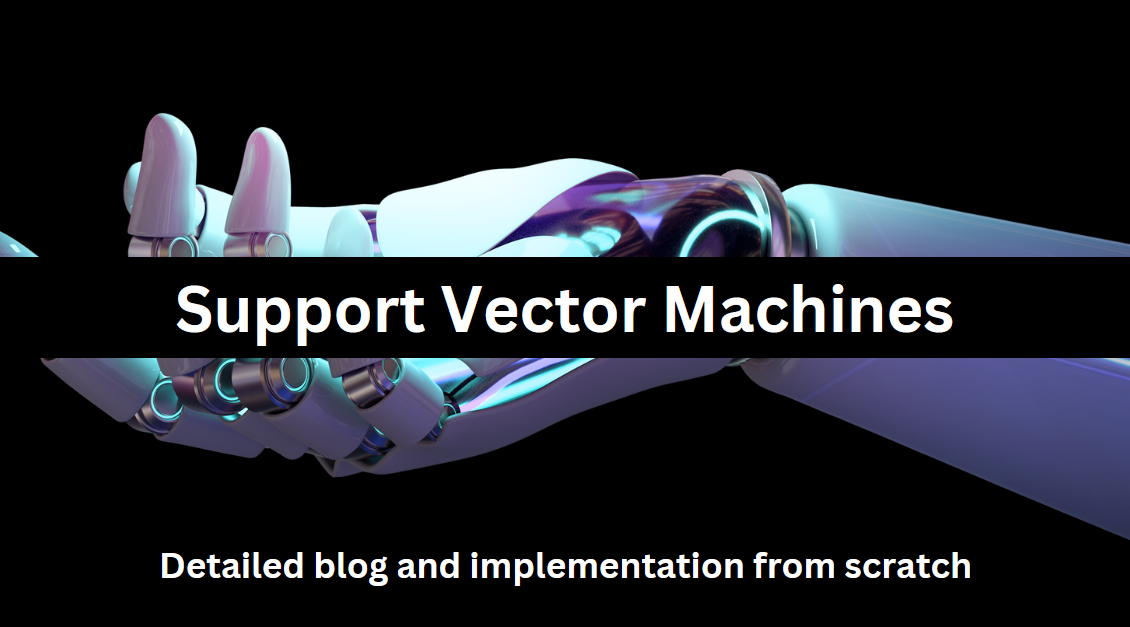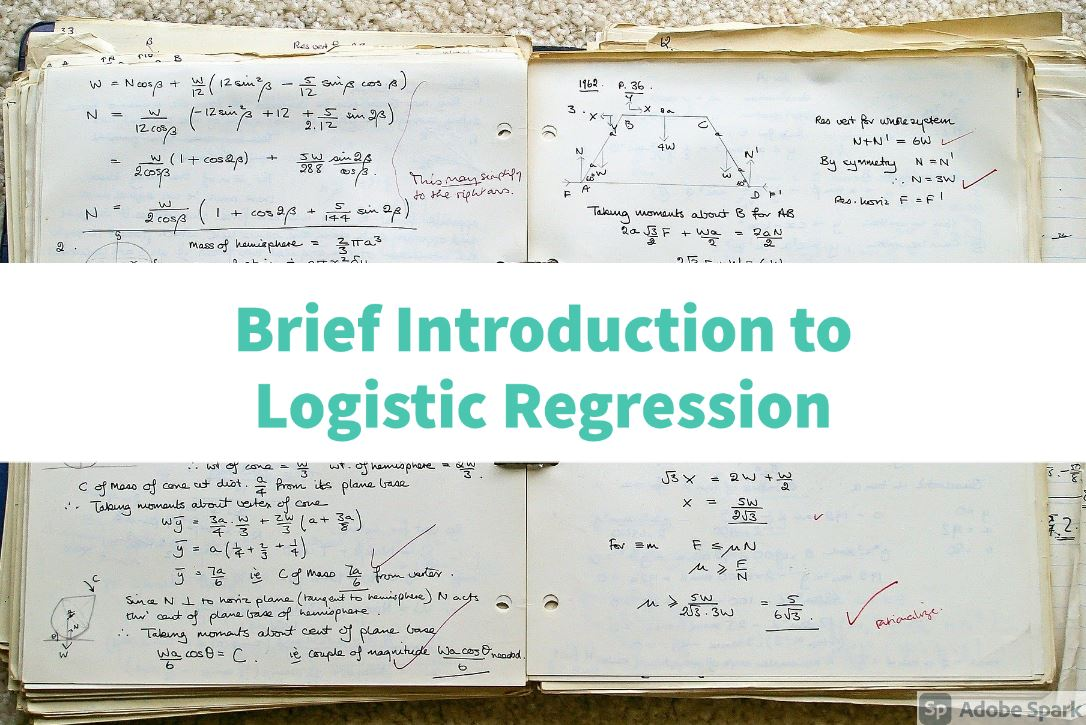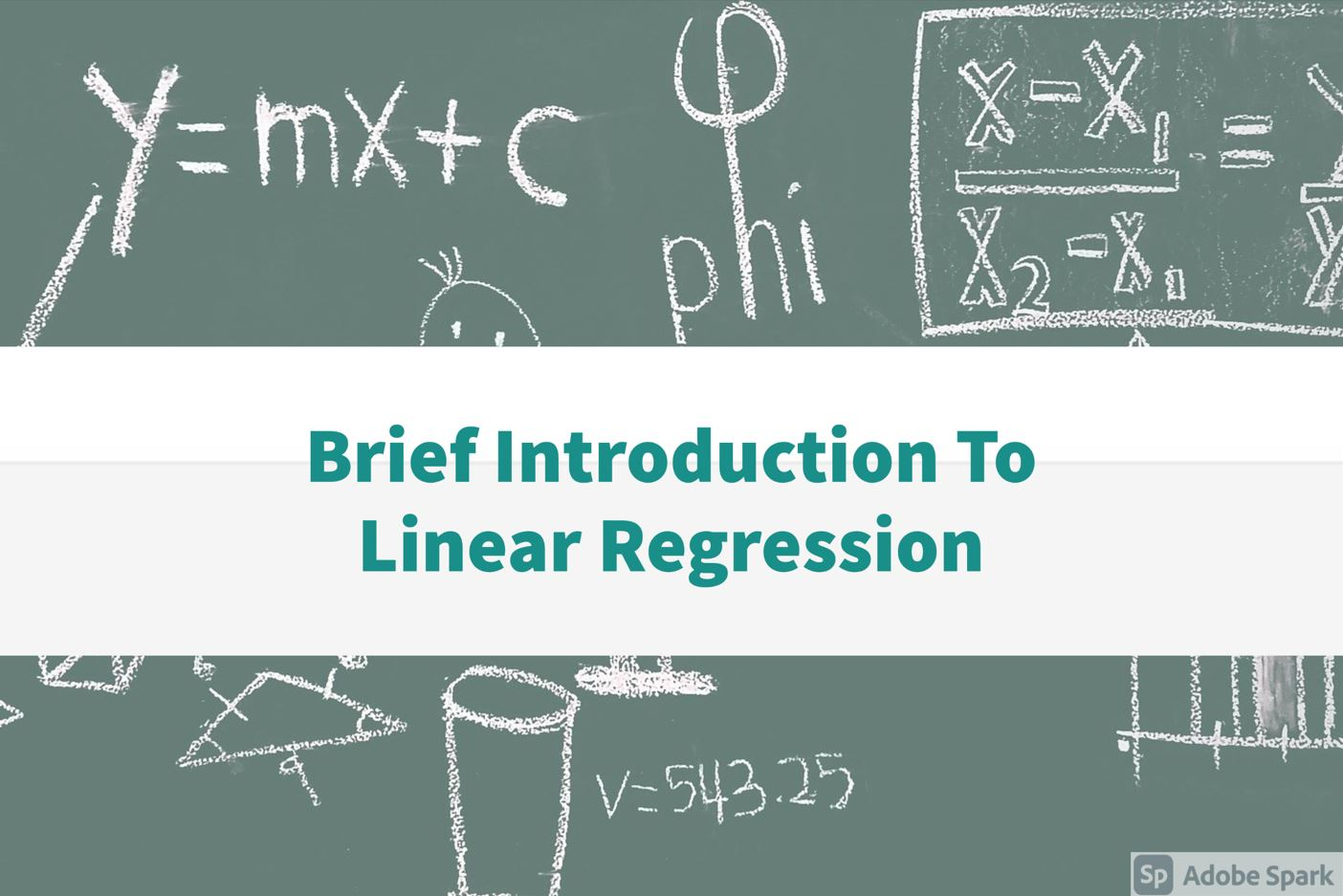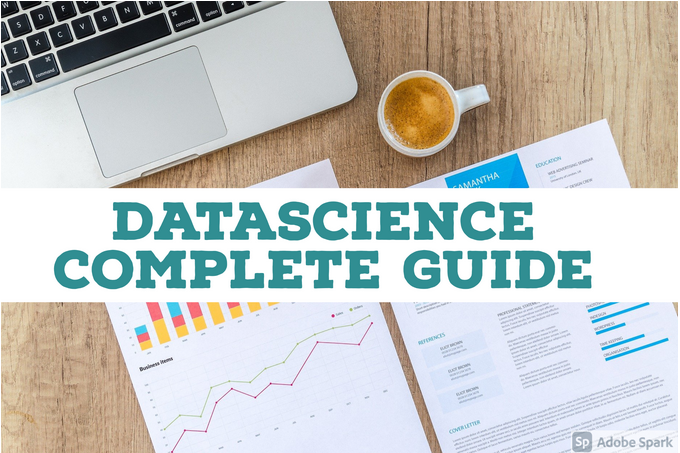# Exploring the Frontiers of AI: A Conversation between ChatGPT and HuggingChat

May 11, 2023, 3:30 p.m.

Artificial intelligence (AI) has been making incredible strides in recent years, and the possibilities of what it can achieve are seemingly limitless. But what are the frontiers of AI, and what lies beyond them? To answer this question, we recently had a fascinating conversation with ChatGPT, a lar…# Support Vector Machines (SVMs) | Complete guide support vector machine algorithm - Python Ocean

Jan. 29, 2023, 7:10 p.m.

SVMs are a type of supervised learning algorithm that can be used for classification and regression tasks. The basic idea behind SVMs is to find a linear boundary (or hyperplane) that separates the data points of different classes in a high-dimensional feature space. The boundary is chosen so that …# Introduction to Decision Tree(with sklearn code)

Feb. 8, 2022, 5:34 p.m.

Decision tree algorithm is one of the most popular machine learning algorithms. It uses tree-like structures and their possible combinations to solve specific problems. It belongs to the category of supervised learning algorithms and can be used for classification and regression purposes. A deci…# An Introduction to Logistic Regression Pythonocean

Oct. 19, 2021, 1:47 p.m.

In this blog, we will talk about the basic concepts of logistics regression and what kind of problems it can help us solve. Logistic Regression is a ranking algorithm used to assign observations to a separate set of classes. Some examples of classification issues are email spam or not spam, online…# Linear Regression Using Gradient Descent Python

Aug. 26, 2021, 10:22 a.m.

Simple Linear regression is one of the simplest and is going to be first AI algorithm which you will learn in this blog.In statistics, a simple linear regression is a linear regression model with a single defining variable. That is, it relates to two-dimensional sample points with an independent va…